How Cheenta works to ensure student success?
Explore the Back-Story

# Test of Mathematics Solution Subjective 176 - Value of a Polynomial at x = n+1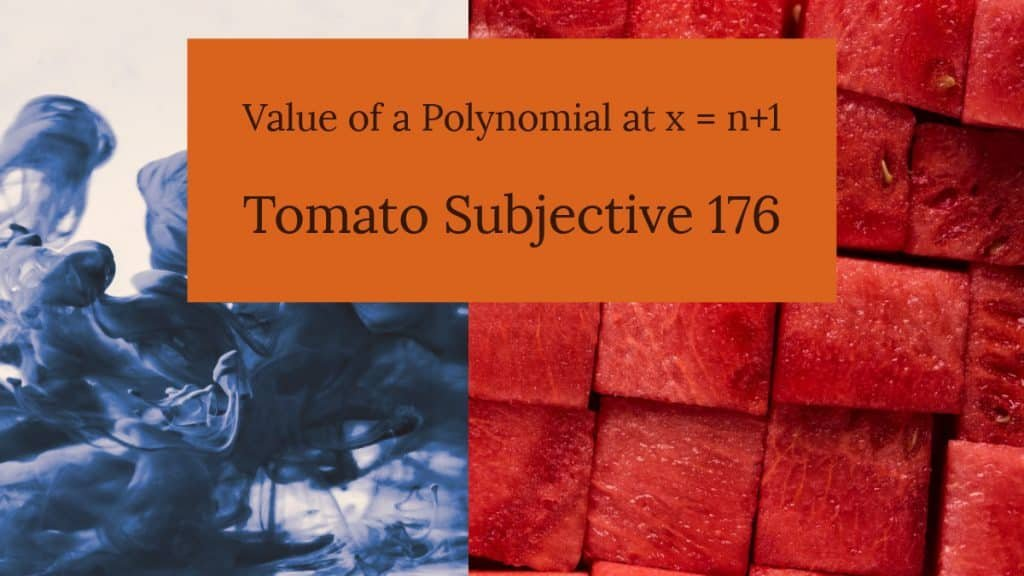This is a Test of Mathematics Solution Subjective 176 (from ISI Entrance). The book, Test of Mathematics at 10+2 Level is Published by East West Press. This problem book is indispensable for the preparation of I.S.I. B.Stat and B.Math Entrance.

Also visit: I.S.I. & C.M.I. Entrance Course of Cheenta

## Problem

Suppose that P(x) is a polynomial of degree n such that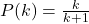for k = 0, 1, 2, ..., n . Find the value of P(n+1).

## Solution

Consider an auxiliary polynomial g(x) = (x+1)P(x) - x . g(x) is an n+1 degree polynomial (as P(x) is n degree and we multiply (x+1) with it). We note that g(0) = g(1) = ... = g(n) = 0  (as the given condition allows (k+1) P(k) - k = 0 for all k from 0 to n). Hence 0, 1, 2, ... , n are the n+1 roots of g(x).

Therefore we may write g(x) = (x+1)P(x) - x = C(x)(x-1)(x-2)...(x-n) where C is a constant. Put x = -1. We get g(-1) = (-1+1)P(-1) - (-1) = C(-1)(-1-1)(-1-2)...(-1-n).

Thus 1 = C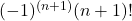gives us the value of C. We put the value of C in the equation (x+1)P(x) - x = C(x)(x-1)(x-2)...(x-n) and replace x by n+1 to get the value of P(n+1).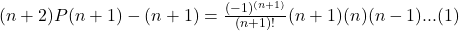implying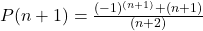This is a Test of Mathematics Solution Subjective 176 (from ISI Entrance). The book, Test of Mathematics at 10+2 Level is Published by East West Press. This problem book is indispensable for the preparation of I.S.I. B.Stat and B.Math Entrance.

Also visit: I.S.I. & C.M.I. Entrance Course of Cheenta

## Problem

Suppose that P(x) is a polynomial of degree n such thatfor k = 0, 1, 2, ..., n . Find the value of P(n+1).

## Solution

Consider an auxiliary polynomial g(x) = (x+1)P(x) - x . g(x) is an n+1 degree polynomial (as P(x) is n degree and we multiply (x+1) with it). We note that g(0) = g(1) = ... = g(n) = 0  (as the given condition allows (k+1) P(k) - k = 0 for all k from 0 to n). Hence 0, 1, 2, ... , n are the n+1 roots of g(x).

Therefore we may write g(x) = (x+1)P(x) - x = C(x)(x-1)(x-2)...(x-n) where C is a constant. Put x = -1. We get g(-1) = (-1+1)P(-1) - (-1) = C(-1)(-1-1)(-1-2)...(-1-n).

Thus 1 = Cgives us the value of C. We put the value of C in the equation (x+1)P(x) - x = C(x)(x-1)(x-2)...(x-n) and replace x by n+1 to get the value of P(n+1).implying### Knowledge Partner